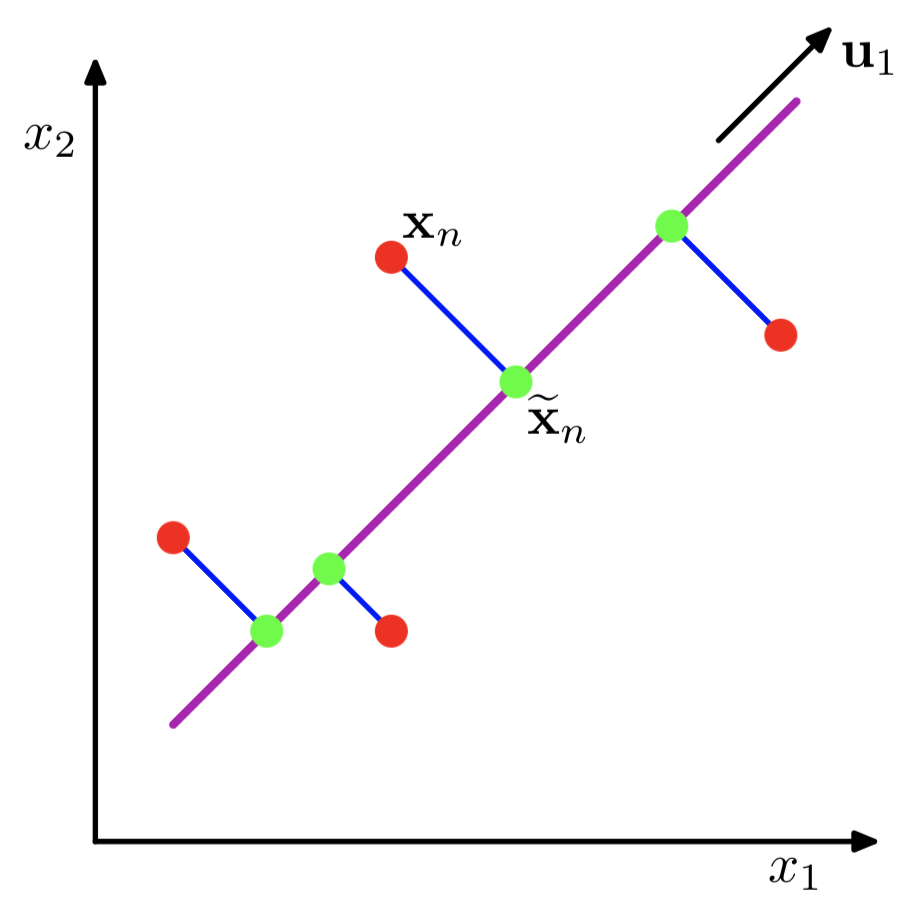【阅读时间】
【内容简介】

# What & Why PCA（主成分分析）

PCA，Principal components analyses，主成分分析。广泛应用于降维，有损数据压缩，特征提取和数据可视化。也被称为Karhunen-Loeve变换

• 最大化正交投影后数据的方差（让数据在经过变换后更加分散PCA定义为使绿色点集的方差最小（方差是尽量让绿色所有点都聚在一坨

• 最小化投影造成的损失（下图中所有红线（投影造成的损失）加起来最小）

PCA 主成分分析主要目的是为了减少数据维数，其中Auto-encoder也是一种精巧的降维手段

# What & Why SVD（奇异值分解）

SVD，Singular Value Decomposition，奇异值分解。最直观的解释如下图所示$$\mathbf M = \mathbf U \cdot \mathbf \Sigma \cdot \mathbf V^*$$

$\mathbf M$ 是一个m×n阶矩阵（输入为n维向量，输出为m维向量

$\mathbf U$ 的列组成一套基向量，m×m阶矩阵，为$\mathbf M \mathbf M^*$ 的特征向量

$\mathbf \Sigma$ 对角矩阵，对角线上的值称为奇异值，可视为在输入与输出之间进行的标量的“伸缩尺度控制”。为 $\mathbf M \mathbf M^*$ 或 $\mathbf M^* \mathbf M$ 的非零特征值的平方根

$\mathbf V^*$ 是 $\mathbf V$ 的共轭转置（实数域即 $\mathbf V^T$），n×n阶矩阵，$\mathbf V$ 的列组成一套基向量，为 $\mathbf M^* \mathbf M$ 的特征向量